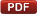## Introduction to programming in Fortran 77

This page contains the study material that I originally prepared for the course MSE 460 Computational Materials Science at the University of Pennsylvania (lecturer: Prof. Vaclav Vitek). If you have absolutely no experience with Fortran, it should help you get started and provide you with basic source of information about programming, specifically in Fortran 77. This is now rather obsolete but understanding this old standard will help you comprehend many older codes that are still used nowadays for calculations.

Here is the main text in PDF or as a HTML web site:Introduction to programming in Fortran 77 for students of Science and Engineering

If you work with Windows, the following link might be of interest for you. It contains various hints to customize your Emacs like e.g. change the keybindings, colors, font, etc.
http://www.gnu.org/software/emacs/windows

Did you try Emacs for MacOS X in the command line terminal ? If you don't like it, have a look at http://www.webweavertech.com/ovidiu/emacs.htm to learn about Emacs which runs entirely as a native Mac application.

If you don't like using Matlab, Maple or Mathematica for plotting your data, consider using the GnuPlot. It is a command-line plotting program which is very popular among scientists. Check the following web site to learn more: http://www.cs.uni.edu/Help/gnuplot
A particularly readable introduction to gnuplot that is very useful also as a further reference can be found at: http://t16web.lanl.gov/Kawano/gnuplot/index-e.html

How can I generate random numbers in F77 ?
F77 provides an intrinsic function called RAND which gives a number from a pseudorandom sequence. If the input parameter is 1, the random sequence generator is restarted. If you give it 0, the function returns a number from the pseudorandom sequence. This is what you need to get a random number in num:
```      num = RAND(0)
```
Note that if you call RAND(1) and then you read the random numbers using RAND(0), you always get the same sequence because the random number generator in Fortran gives really something what people call "pseudorandom" numbers. If you give RAND other number than 0 or 1, it uses this number as a seed for the generation of the random sequence (if you want to learn details, check with some advanced math book).
The atoms in my block are plotted in Matlab as empty circles. How can I draw them filled ?
Most likely, the plotting of your atomic structure is done using the Matlab command plot. This command always returns a handle (or a pointer) to the properties of your plot. For example:
```      handle = plot(x,y,'ko');
```
plots the block of atoms with coordinates stored in two vectors x and y such that each atom is represented by a little black circle. Then, you just have to change the property of the circle to make it filled. Write this line below your plot command:
```      set(handle,'MarkerFaceColor','k');
```
This sets up black as the color which will be used to fill the circles.
The do-while loop does not work.
There is an error in my introductory text to programming in F77 above (thanks Aimee). The correct structure of the do-while loops is:
```      do while (condition)
:
:
enddo
```
The loop is being executed as long as the condition is true. Once it becomes false, the program continues below enddo. An updated version (at least 1.2) of the introductory manual is already given above.
The syntax of my Fortran code is not displayed in color.
By default, Emacs for Windows and Mac has this coloring switched off. To turn it off, create in the c:\ directory (Windows) or your home directory (Mac) a file .emacs (don't forget to include the comma) and copy the following lines into it.
```(cond ((fboundp 'global-font-lock-mode)
;; Turn on font-lock in all modes that support it
(global-font-lock-mode t)
;; Maximum colors
(setq font-lock-maximum-decoration t)))
```
Then, save the file and restart Emacs.